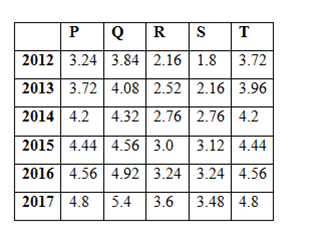# RRB Clerk Prelims Quantitative Aptitude (Day-05)

Dear Aspirants, Our IBPS Guide team is providing new series of Quantitative Aptitude Questions for RRB Clerk 2020 Prelims so the aspirants can practice it on a daily basis. These questions are framed by our skilled experts after understanding your needs thoroughly. Aspirants can practice these new series questions daily to familiarize with the exact exam pattern and make your preparation effective.

Start Quiz

Ensure Your Ability Before the Exam Take IBPS RRB Clerk 2020 Prelims Free Mock Test

Data Interpretation

Directions (1 – 5) Study the following information carefully and answer the given questions:

Following table shows the amount (In lakhs) earned by 5 persons in 6 different years.1) The total amount earned by all the given persons in the year 2012 and 2014 together is approximately what percentage more/less than the total amount earned by all the given persons in the year 2016 and 2017 together?

A) 42 % more

B) 37 % less

C) 23 % less

D) 51 % more

E) 12 % less

2) Find the difference between the total amount earned by Q in all the given years together to that of total amount earned by S in all the given years together?

A) 10.56 lakhs

B) 12.34 lakhs

C) 8.78 lakhs

D) 9.22 lakhs

E) None of these

3) Find the ratio between the total amount earned by P, Q and R in the year 2012, 2014 and 2016 together to that of total amount earned by R, S and T in the year 2013, 2015 and 2017 together?

A) 132 : 119

B) 215 : 176

C) 345 : 247

D) 277 : 259

E) None of these

4) Find the average amount earned by P, R and T together in all the given years?

A) 19.36 lakhs

B) 17.58 lakhs

C) 22.64 lakhs

D) 25.72 lakhs

E) None of these

5) The total amount earned by Q in the year 2012, 2014 and 2016 together is approximately what percentage of total amount earned by S in the year 2013, 2015 and 2017 together?

A) 150 %

B) 130 %

C) 115 %

D) 85 %

E) 165 %

Approximation

Directions (6 – 10): What approximate value should come in place of the question mark (?) in the following questions? (You are not expected to calculate the exact value.)

6)  (26/9)2 × (399/39) ÷ (41/899) = ?

A) 2070

B) 1800

C) 1700

D) 2550

E) 1950

7) – (4.99)3 + (29.98)2 – (3.01)4 = ?

A) 550

B) 590

C) 620

D) 650

E) 690

8) [(7.99)2 – (13.001)2 + (4.01)3]2 = ?

A) -1800

B) 1450

C) -1680

D) 1680

E) -1450

9) √(675.001) + (4.005)= ?

A) 84

B) 86

C) 90

D) 94

E) 87

10) √(727.99950) + (5.1961)2 = ? ÷ (2/10.7960)

A) 30

B) 25

C) 5

D) 15

E) 10

Directions (1-5) :

The total amount earned by all the given persons in the year 2012 and 2014 together

= > 3.24 + 3.84 + 2.16 + 1.8 + 3.72 + 4.2 + 4.32 + 2.76 + 2.76 + 4.2

= > 33 lakhs

The total amount earned by all the given persons in the year 2016 and 2017 together

= > 4.56 + 4.92 + 3.24 + 3.24 + 4.56 + 4.8 + 5.4 + 3.6 + 3.48 + 4.8

= > 42.6 lakhs

Required % = [(42.6 – 33)/42.6]*100 = 22.53 % = 23 % less

The total amount earned by Q in all the given years together

= > 3.84 + 4.08 + 4.32 + 4.56 + 4.92 + 5.4

= > 27.12 lakhs

The total amount earned by S in all the given years together

= > 1.8 + 2.16 + 2.76 + 3.12 + 3.24 + 3.48

= > 16.56 lakhs

Required difference = 27.12 – 16.56 = 10.56 lakhs

The total amount earned by P, Q and R in the year 2012, 2014 and 2016 together

= > 3.24 + 3.84 + 2.16 + 4.2 + 4.32 + 2.76 + 4.56 + 4.92 + 3.24

= > 33.24 lakhs

The total amount earned by R, S and T in the year 2013, 2015 and 2017 together

= > 2.52 + 2.16 + 3.96 + 3.0 + 3.12 + 4.44 + 3.6 + 3.48 + 4.8

= > 31.08 lakhs

Required ratio = 33.24: 31.08 = 277: 259

The total amount earned by P, R and T together in all the given years

= > 3.24 + 3.72 + 4.2 + 4.44 + 4.56 + 4.8 + 2.16 + 2.52 + 2.76 + 3.0 + 3.24 + 3.6 + 3.72 + 3.96 + 4.2 + 4.44 + 4.56 + 4.8

= > 67.92 lakhs

Required average = 67.92/3 = 22.64 lakhs

The total amount earned by Q in the year 2012, 2014 and 2016 together

= > 3.84 + 4.32 + 4.92 =13.08 lakhs

The total amount earned by S in the year 2013, 2015 and 2017 together

= > 2.16 + 3.12 + 3.48 = 8.76 lakhs

Required % = (13.08/8.76)*100 = 149.31 % = 149 % = 150 %

Directions (6-10) :

x = (27/9)2 × (400/40) ÷ (40/900)

x = 32 × (400/40) × (900/40)

x = 9 × 10 × 23 = 2070

?= – (4.99)3 + (29.98)2 – (3.01)4

=> ? = – (5)3 + (30)2 – (3)4

=> ? = – 125 + 900 – 81

=> ? = 900 – 206

=> ? = 694

=> ? = 690 (approx.)

[(7.99)2 – (13.001)2 + (4.01)3]2

=> [(8)2 – (13)2 + (4)3]2 = ?

=> [64 – 169 + 64]2 = ?

=> (-41)2 = ?

? = 1681 ≈1680

√(675.001) + (4.005)3 = ?

=> ? ≈ √(26×26) + (4)3

= 26 + 64 = 90

√(727.9995) + (5.1961)2 = ? ÷ (2/10.7960)

=> √729 + 52 ≈ ? ÷ (2/11)

=> 27 + 25 = ? ÷ (2/11)

=> 52 × (2/11) = ? ≈ 10

 Check Here to View RRB Clerk Prelims 2020 Quantitative Aptitude Questions Day – 04 Day – 03 Day – 02